### 一.图像顶帽运算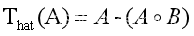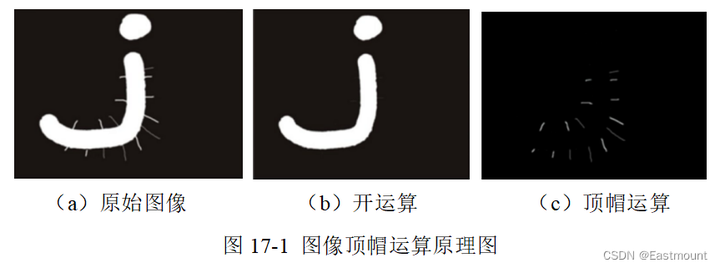dst = cv2.morphologyEx(src, cv2.MORPH_TOPHAT, kernel)

• src表示原始图像
• cv2.MORPH_TOPHAT表示图像顶帽运算
• kernel表示卷积核，可以用numpy.ones()函数构建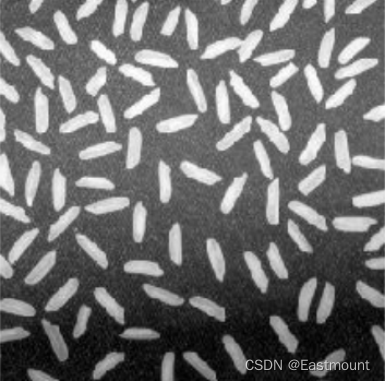``````# -*- coding: utf-8 -*-
# By：Eastmount
import cv2
import numpy as np
#读取图片
#设置卷积核
kernel = np.ones((10,10), np.uint8)
#图像顶帽运算
result = cv2.morphologyEx(src, cv2.MORPH_TOPHAT, kernel)
#显示图像
cv2.imshow("src", src)
cv2.imshow("result", result)
#等待显示
cv2.waitKey(0)
cv2.destroyAllWindows()``````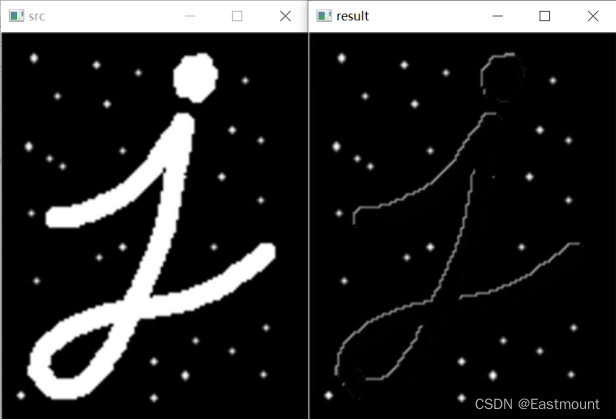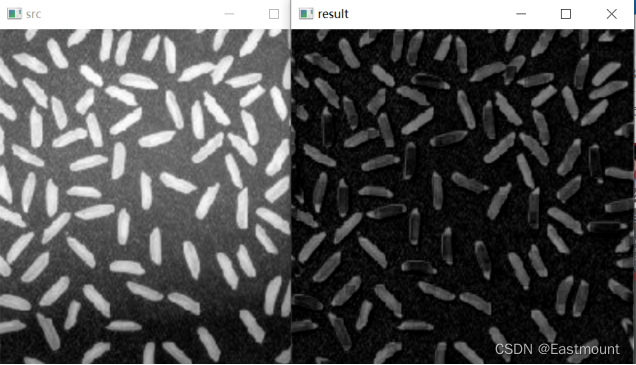``````# -*- coding: utf-8 -*-
# By：Eastmount
import numpy as np
import cv2 as cv
import matplotlib.pyplot as plt
from mpl_toolkits.mplot3d import Axes3D
from matplotlib import cm
from matplotlib.ticker import LinearLocator, FormatStrFormatter
#读取图像
img = cv.cvtColor(img,cv.COLOR_BGR2GRAY)
imgd = np.array(img) #image类转numpy
#准备数据
sp = img.shape
h = int(sp) #图像高度(rows)
w = int(sp) #图像宽度(colums) of image
#绘图初始处理
fig = plt.figure(figsize=(16,12))
ax = fig.gca(projection="3d")
x = np.arange(0, w, 1)
y = np.arange(0, h, 1)
x, y = np.meshgrid(x,y)
z = imgd
surf = ax.plot_surface(x, y, z, cmap=cm.coolwarm)
#自定义z轴
ax.set_zlim(-10, 255)
ax.zaxis.set_major_locator(LinearLocator(10)) #设置z轴网格线的疏密
#将z的value字符串转为float并保留2位小数
ax.zaxis.set_major_formatter(FormatStrFormatter('%.02f'))
# 设置坐标轴的label和标题
ax.set_xlabel('x', size=15)
ax.set_ylabel('y', size=15)
ax.set_zlabel('z', size=15)
ax.set_title("surface plot", weight='bold', size=20)
#添加右侧的色卡条
fig.colorbar(surf, shrink=0.6, aspect=8)
plt.show()``````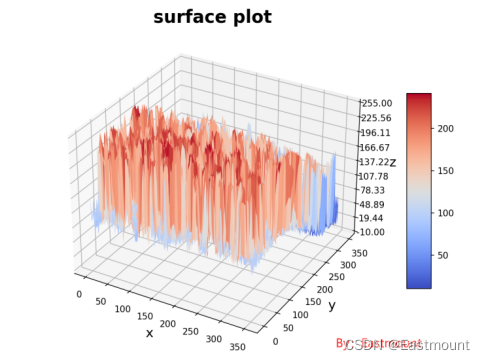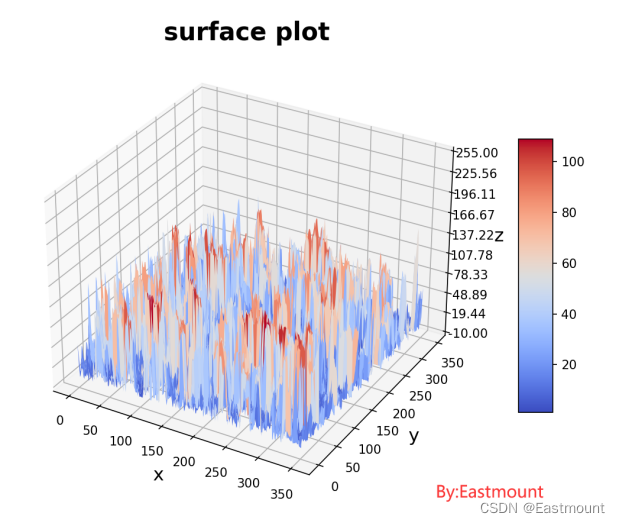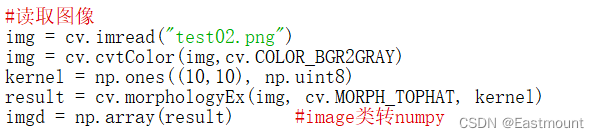### 二.图像底帽运算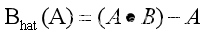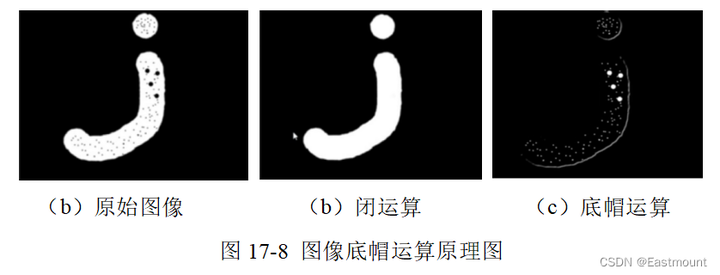dst = cv2.morphologyEx(src, cv2.MORPH_BLACKHAT, kernel)

• src表示原始图像
• cv2.MORPH_BLACKHAT表示图像底帽或黑帽运算
• kernel表示卷积核，可以用numpy.ones()函数构建

Python实现图像底帽运算的代码如下所示：

``````# -*- coding: utf-8 -*-
# By：Eastmount
import cv2
import numpy as np
#读取图片
#设置卷积核
kernel = np.ones((10, 10), np.uint8)
#图像黑帽运算
result = cv2.morphologyEx(src, cv2.MORPH_BLACKHAT, kernel)
#显示图像
cv2.imshow("src", src)
cv2.imshow("result", result)
#等待显示
cv2.waitKey(0)
cv2.destroyAllWindows()``````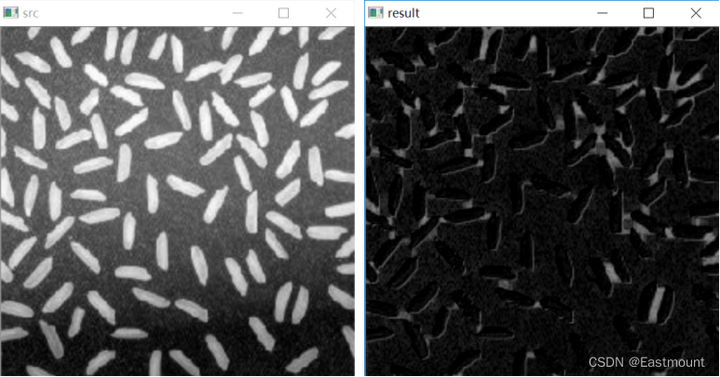### 参考文献：

• 冈萨雷斯著，阮秋琦译. 数字图像处理（第3版）[M]. 北京：电子工业出版社，2013.
• 阮秋琦. 数字图像处理学（第3版）[M]. 北京：电子工业出版社，2008.
• 毛星云，冷雪飞. OpenCV3编程入门[M]. 北京：电子工业出版社，2015.
• Eastmount. [Python图像处理] 八.图像腐蚀与图像膨胀[EB/OL]. (2018-10-31). https://blog.csdn.net/Eastmount/article/details/83581277.